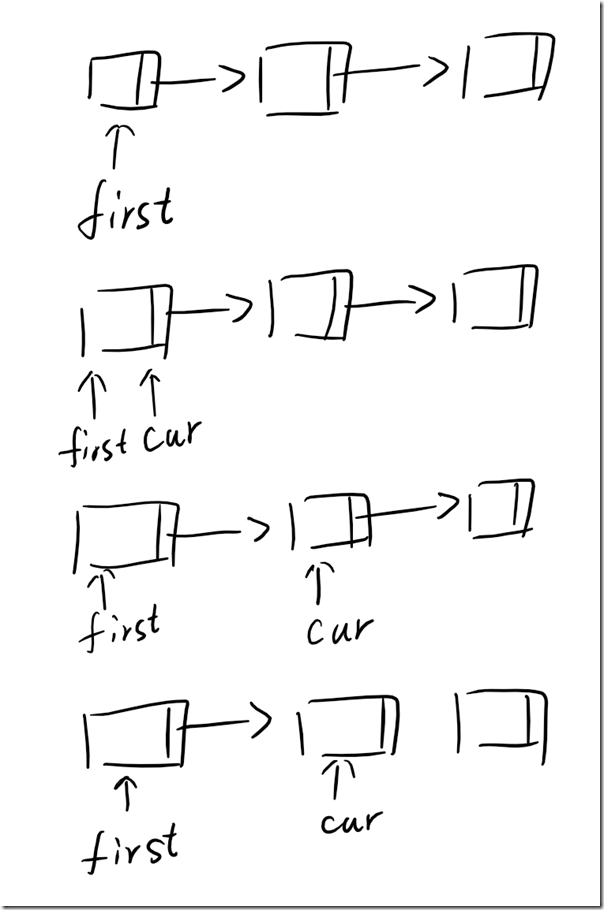1.3.19

# 1.3.19 #

## 解答 ### 代码 #

var first = new Node<string> { Item = "first" };
var second = new Node<string> { Item = "second" };
var third = new Node<string> { Item = "third" };

first.Next = second;
second.Next = third;
third.Next = null;

var current = first;
while (current != null)
{
Console.Write(current.Item + " ");
current = current.Next;
}

DeleteLast(first);
Console.WriteLine();

current = first;
while (current != null)
{
Console.Write(current.Item + " ");
current = current.Next;
}

Console.WriteLine();

static void DeleteLast(Node<string> first)
{
var current = first;

while (current.Next.Next != null)
{
current = current.Next;
}

current.Next = null;
}


Generics 库